International
Tables for
Crystallography
Volume A
Space-group symmetry
Edited by M. I. Aroyo

International Tables for Crystallography (2016). Vol. A, ch. 2.1, pp. 160-161

## Section 2.1.3.9. Symmetry operations

Th. Hahna and A. Looijenga-Vosb

#### 2.1.3.9. Symmetry operations

| top | pdf |

As explained in Sections 1.3.3.2and 1.4.2.3, the coordinate triplets of the General position of a space group may be interpreted as a shorthand description of the symmetry operations in matrix notation. The geometric description of the symmetry operations is found in the space-group tables under the heading Symmetry operations.

Numbering scheme. The numbering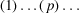of the entries in the blocks Symmetry operations and General position (first block below Positions) is the same. Each listed coordinate triplet of the general position is preceded by a number between parentheses (p). The same number (p) precedes the corresponding symmetry operation. For space groups with primitive cells, the two lists contain the same number of entries.

For space groups with centred cells, several (2, 3 or 4) blocks of Symmetry operations correspond to the one General position block. The numbering scheme of the general position is applied to each one of these blocks. The number of blocks equals the multiplicity of the centred cell, i.e. the number of centring translations below the subheading Coordinates, such as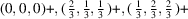.

Whereas for the Positions the reader is expected to add these centring translations to each printed coordinate triplet themselves (in order to obtain the complete general position), for the Symmetry operations the corresponding data are listed explicitly. The different blocks have the subheadings For (0, 0, 0)+ set', For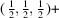set', etc. Thus, an obvious one-to-one correspondence exists between the analytical description of a symmetry operation in the form of its general-position coordinate triplet and the geometrical description under Symmetry operations. Note that the coordinates are reduced modulo 1, where applicable, as shown in the example below.

#### Example: Ibca (73)

The centring translation is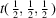. Accordingly, above the general position one findsand. In the block Symmetry operations, under the subheading Forset', entry (2) refers to the coordinate triplet. Under the subheading Forset', however, entry (2) refers to. The tripletis selected rather than, because the coordinates are reduced modulo 1.

The coordinate triplets of the general position represent the symmetry operations chosen as coset representatives of the decomposition of the space group with respect to its translation subgroup (cf. Section 1.4.2for a detailed discussion). In space groups with two origins the origin shift may lead to the choice of symmetry operations of different types as coset representatives of the same coset (e.g. mirror versus glide plane, rotation versus screw axis, see Tables 1.4.2.2and 1.4.2.3) and designated by the same number (p) in the general-position blocks of the two descriptions. Thus, in(129), (p) = (7) represents a 2 and a 21 axis, both in, whereas (p) = (16) represents a g and an m plane, both in.

Designation of symmetry operations. An entry in the block Symmetry operations is characterized as follows.

 (i) A symbol denoting the type of the symmetry operation (cf. Section 2.1.2), including its glide or screw part, if present. In most cases, the glide or screw part is given explicitly by fractional coordinates between parentheses. The sense of a rotation is indicated by the superscriptor −. Abbreviated notations are used for the glide reflections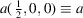;;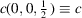. Glide reflections with complicated and unconventional glide parts are designated by the letter g, followed by the glide part between parentheses. (ii) A coordinate triplet indicating the location and orientation of the symmetry element which corresponds to the symmetry operation. For rotoinversions, the location of the inversion point is also given.

#### Examples

 (1)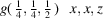Glide reflection with glide component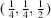through the plane x, x, z, i.e. the plane parallel tocontaining the point 0, 0, 0. (2)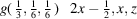(hexagonal axes) Glide reflection with glide component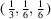through the plane, i.e. the plane parallel to, which intersects the a axis atand the b axis at; this operation occurs in(167, hexagonal axes). (3) Symmetry operations in Ibca (73) Under the subheading For (0, 0, 0)set', the operation generating the coordinate triplet (2)from (1) x, y, z is symbolized by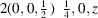. This indicates a twofold screw rotation with screw part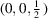for which the corresponding screw axis coincides with the line, i.e. runs parallel to  through the point. Under the subheading Forset', the operation generating the coordinate triplet (2)from (1) x, y, z is symbolized by. It is thus a twofold rotation (without screw part) around the line.

Details on the symbolism and further illustrative examples are presented in Section 1.4.2.1.